Hyperlink & Search Engine Law News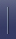Decisions & Court Documents WorldwideLinking Law CasesSearch Engine LawTechnical    Background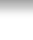PageRank

PageRank is a family of algorithms for assigning numerical weightings to hyperlinked documents (or web pages) indexed by a search engine. Its properties are much discussed by search engine optimization (SEO) experts. The PageRank system is used by the popular search engine Google to help determine a page's relevance or importance. It was developed by Google's founders Larry Page and Sergey Brin while at Stanford University in 1998. As Google puts it (http://www.google.com/technology/):

PageRank relies on the uniquely democratic nature of the web by using its vast link structure as an indicator of an individual page's value. Google interprets a link from page A to page B as a vote, by page A, for page B. But, Google looks at more than the sheer volume of votes, or links a page receives; it also analyzes the page that casts the vote. Votes cast by pages that are themselves "important" weigh more heavily and help to make other pages "important."

In other words, a page rank results from a "ballot" among all the other pages on the World Wide Web about how important a page is. A hyperlink to a page counts as a vote of support. The PageRank of a page is defined recursively and depends on the number and PageRank metric of all pages that link to it ("incoming links"). A page that is linked by many pages with high rank receives a high rank itself. If there are no links to a web page there is no support of this specific page. The Google Toolbar PageRank goes from 0 to 10. It seems to be a logarithmic scale. The exact details of this scale are unknown.

The name PageRank is a trademark of Google. Whether or not the pun on the name Larry Page and the word "page" was intentional or accidental remains an open question. The PageRank process has been patented ( U.S. Patent 6,285,999 (http://patft.uspto.gov/netacgi/nph-Parser?Sect1=PTO1&Sect2=HITOFF&d=PALL&p=1&u=/netahtml/srchnum.htm&r=1&f=G&l=50&s1=6,285,999.WKU.&OS=PN/6,285,999&RS=PN/6,285,999)).

An alternative to the Page rank algorithm proposed by Jon Kleinberg is the HITS algorithm.

## Page rank algorithm

### Simplified

Suppose a small universe of four web pages: A, B, C and D. If all those pages link to A, then the PR (PageRank) of page A would be the sum of the PR of pages B, C and D.

PR(A) = PR(B) + PR(C) + PR(D)

But then suppose page B also has a link to page C, and page D has links to all three pages. One cannot vote twice, and for that reason it is considered that page B has given half a vote to each. In the same logic, only one third of D's vote is counted for A's PageRank.$PR(A)= \frac{PR(B)}{2}+ \frac{PR(C)}{1}+ \frac{PR(D)}{3}$

In other words, divide the PR by the total number of links that come from the page.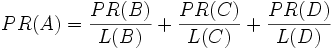$PR(A)= \frac{PR(B)}{L(B)}+ \frac{PR(C)}{L(C)}+ \frac{PR(D)}{L(D)}$

Finally, all of this is reduced by a certain percentage by multiplying it by a factor q. For reasons explained below, no page can have a PageRank of 0. As such, Google performs a mathematical operation and gives everyone a minimum of 1 - q. It means that if you reduced 15% everyone you give them back 0.15.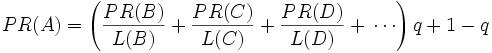$PR(A)=\left( \frac{PR(B)}{L(B)}+ \frac{PR(C)}{L(C)}+ \frac{PR(D)}{L(D)}+\,\cdots \right) q + 1 - q$

So one page's PageRank is calculated by the PageRank of other pages. Google is always recalculating the PageRanks. If you give all pages a PageRank of any number (except 0) and constantly recalculate everything, all PageRanks will change and tend to stabilize at some point. It is at this point where the PageRank is used by the search engine.

### Complex

The formula uses a model of a random surfer who gets bored after several clicks and switches to a random page. The PageRank value of a page reflects the frequency of hits on that page by the random surfer. It can be understood as a Markov process in which the states are pages, and the transitions are all equally probable and are the links between pages. If a page has no links to another pages, it becomes a sink and therefore makes this whole thing unusable, because the sink pages will trap the random visitors forever. However, the solution is quite simple. If the random surfer arrives to a sink page, it picks another URL at random and continues surfing again.

To be fair with pages that are not sinks, these random transitions are added to all nodes in the Web, with a residual probability of usually q=0.15, estimated from the frequency that an average surfer uses his or her browser's bookmark feature.

So, the equation is as follows: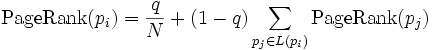${\rm PageRank}(p_i) = \frac{q}{N} + (1 -q) \sum_{p_j \in L(p_i)} {\rm PageRank} (p_j)$

where p1,p2,...,pN are the pages under consideration, L(pi) is the set of pages that link to pi, and N is the total number of pages.

The PageRank values are the entries of the dominant eigenvector of the modified adjacency matrix. This makes PageRank a particularly elegant metric: the eigenvector is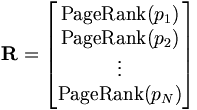$\mathbf{R} = \begin{bmatrix} {\rm PageRank}(p_1) \\ {\rm PageRank}(p_2) \\ \vdots \\ {\rm PageRank}(p_N) \end{bmatrix}$

where R is the solution of the equation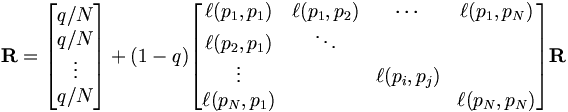$\mathbf{R} = \begin{bmatrix} {q / N} \\ {q / N} \\ \vdots \\ {q / N} \end{bmatrix} + (1-q) \begin{bmatrix} \ell(p_1,p_1) & \ell(p_1,p_2) & \cdots & \ell(p_1,p_N) \\ \ell(p_2,p_1) & \ddots & & \\ \vdots & & \ell(p_i,p_j) & \\ \ell(p_N,p_1) & & & \ell(p_N,p_N) \end{bmatrix} \mathbf{R}$

where the adjacency function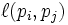$\ell(p_i,p_j)$ is 0 if page pi does not link to pj, and normalised such that, for each i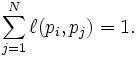$\sum_{j = 1}^N \ell(p_i,p_j) = 1.$

The values of the PageRank eigenvector are fast to approximate (only a few iterations are needed) and in practice it gives good results.

As a result of Markov theory, it can be shown that the PageRank of a page is the probability of being at that page after lots of clicks. This happens to equal t - 1 where t is the expectation of the number of clicks (or random jumps) required to get from the page back to itself.

The main disadvantage is that it favors older pages, because a new page, even a very good one, will not have many links unless it is part of an existing site (a site being a densely connected set of pages).

That's why PageRank should be combined with textual analysis or other ranking methods. PageRank seems to favor Wikipedia pages, often putting them high or at the top of searches for several encyclopedic topics. A common theory is that this is because Wikipedia is very interconnected, with each article having many internal links from other articles, which in turn have links from many other sites on the Web pointing to them. Compared to Wikipedia, and similar high quality content-rich sites, the rest of the World Wide Web is relatively loosely connected.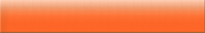Overview of the Section Search Engine, Linking & Framing Terminology Explained!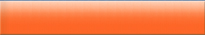Legal trouble for YouTube in Germany